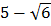# From the tower 60 m high angles of depression of the top and bottom of a house areandrespectively. If the height of the house is, thenis equal to a)b)c)d)## Question ID - 50337 :- From the tower 60 m high angles of depression of the top and bottom of a house areandrespectively. If the height of the house is, thenis equal to a)b)c)d)3537

(d)

In…(i)

In[from Eq.(i)](given)Next Question :
 In a triangle, the lengths of the two larger sides are 10 cm and 9 cm respectively. If the angles of the triangle are in AP, then the length of the third side in cm can be a)only b)only c)ord) Neithernor